CREATOR ONLY

Creating and Manipulating Charts with MatPlotLib

This topic covers everything you need to know about creating a variety of 2D and 3D charts with MatPlotLib.

TECHNOLOGIES

Data Visualization
MatPlotLib

44 minutes

Lessons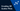Creating 3D Scatter Plots

Learn to create 3D scatter plots with MatPlotLib.

4 min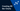Creating 3D Bar Charts

Learn to create 3D bar charts with MatPlotLib.

8 min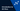Introduction to 3D Plots

Understand the basics about creating 3D charts with MatPlotLib.

6 min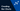Creating Bar Charts

Learn to create bar charts with MatPlotLib.

9 min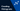Creating Histograms

Learn to create Histograms with MatPlotLib.

7 min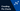Creating Pie Charts

Understand how to create pie charts with MatPlotLib.

8 min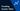Creating Scatter Plots

Understand how to create scatter plots with MatPlotLib.

6 min